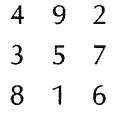# magic square

(redirected from Date magic square)
Also found in: Dictionary, Thesaurus.

## square, magic:

see magic squaremagic square,
a square divided into parts with letters or numbers inscribed therein that, whether combined vertically, horizontally, or diagonally, form the same sum or the same word.
..... Click the link for more information.
.

## magic square,

a square divided into parts with letters or numbers inscribed therein that, whether combined vertically, horizontally, or diagonally, form the same sum or the same word. In ancient times such squares were thought to have magic properties, perhaps connected with the stars. Magic squares have been found in such widely divergent cultures as ancient China, Egypt, and India, as well as W Europe. Example:### Bibliography

See W. S. Andrews, Magic Squares and Cubes (2d ed. 1917, repr. 1960).

The Columbia Electronic Encyclopedia™ Copyright © 2013, Columbia University Press. Licensed from Columbia University Press. All rights reserved. www.cc.columbia.edu/cu/cup/
The following article is from The Great Soviet Encyclopedia (1979). It might be outdated or ideologically biased.

## Magic Square

a square divided into an equal number n of columns and rows with the resulting cells containing the first n2 natural numbers whose sum in each column, each row, and the two main diagonals is the same [being equal, as can be shown, to (½) n(n2 + 1)]. It has been proved that a magic square can be constructed for any n beginning with n = 3. Figure 1, a shows magic squares for n = 3 and n = 4. There exist magic squares that satisfy a number of additional conditions, as for example, a magic square with 64 cells (see Figure 1, b), which can be divided into four smaller squares each containing 16 cells such that the sum of the numbers of any row, any column, or the main diagonals is the same (130). Magic squares were used as talismans in India and some other countries. The construction of magic squares is a classical example of mathematical games and puzzles.Figure 1

### REFERENCE

Postnikov, M. M. Magicheskie kvadraty. Moscow, 1964.
The Great Soviet Encyclopedia, 3rd Edition (1970-1979). © 2010 The Gale Group, Inc. All rights reserved.

## magic square

[′maj·ik ′skwer]
(mathematics)
A square array of integers where the sum of the entries of each row, each column, and each diagonal is the same.
A square array of integers where the sum of the entries in each row and each column (but not necessarily each diagonal) is the same. Also known as semimagic square.
McGraw-Hill Dictionary of Scientific & Technical Terms, 6E, Copyright © 2003 by The McGraw-Hill Companies, Inc.
Site: Follow: Share:
Open / Close